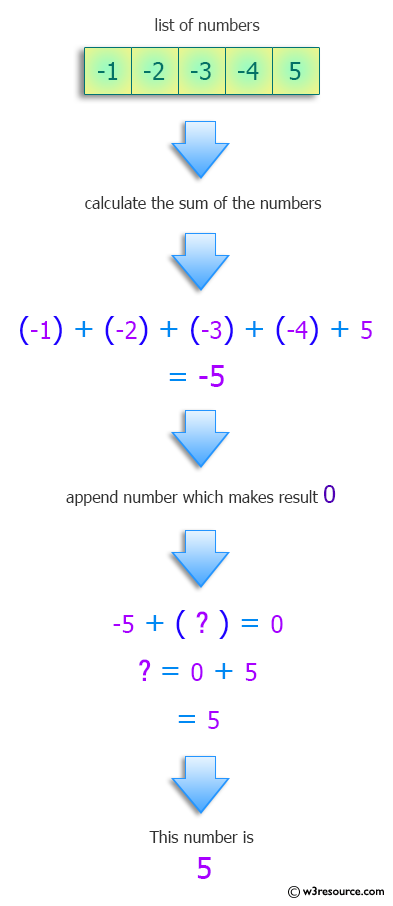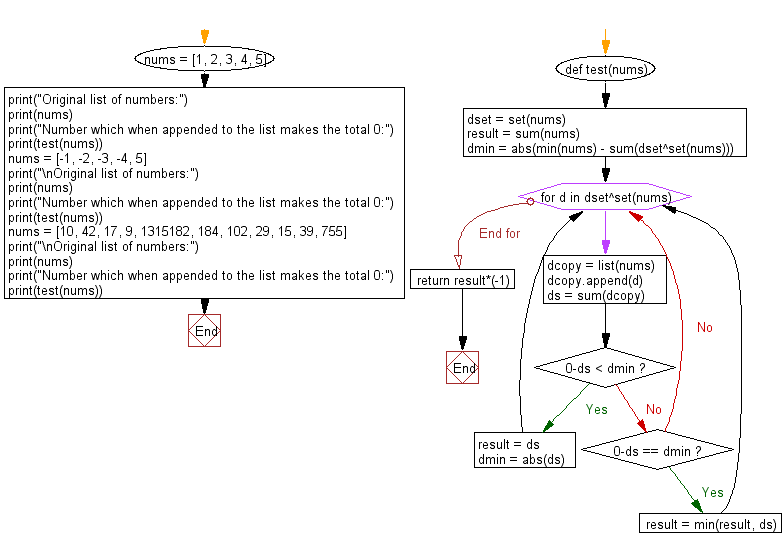﻿ Python: Find the number which when appended to the list makes the total 0 - w3resource# Python: Find the number which when appended to the list makes the total 0

## Python Programming Puzzles: Exercise-61 with Solution

Write a Python program to find the number which when appended to the list makes the total 0.

```Input:
[1, 2, 3, 4, 5]
Output:
-15

Input:
[-1, -2, -3, -4, 5]
Output:
5

Input:
[10, 42, 17, 9, 1315182, 184, 102, 29, 15, 39, 755]
Output:
-1316384
```

Pictorial Presentation:Sample Solution:

Python Code:

``````#License: https://bit.ly/3oLErEI

def test(nums):
dset = set(nums)
result = sum(nums)
dmin = abs(min(nums) - sum(dset^set(nums)))
for d in dset^set(nums):
dcopy = list(nums)
dcopy.append(d)
ds = sum(dcopy)
if 0-ds < dmin:
result = ds
dmin = abs(ds)
elif 0-ds == dmin:
result = min(result, ds)
return result*(-1)

nums = [1, 2, 3, 4, 5]
print("Original list of numbers:")
print(nums)
print("Number which when appended to the list makes the total 0:")
print(test(nums))
nums = [-1, -2, -3, -4, 5]
print("\nOriginal list of numbers:")
print(nums)
print("Number which when appended to the list makes the total 0:")
print(test(nums))
nums = [10, 42, 17, 9, 1315182, 184, 102, 29, 15, 39, 755]
print("\nOriginal list of numbers:")
print(nums)
print("Number which when appended to the list makes the total 0:")
print(test(nums))
``````

Sample Output:

```Original list of numbers:
[1, 2, 3, 4, 5]
Number which when appended to the list makes the total 0:
-15

Original list of numbers:
[-1, -2, -3, -4, 5]
Number which when appended to the list makes the total 0:
5

Original list of numbers:
[10, 42, 17, 9, 1315182, 184, 102, 29, 15, 39, 755]
Number which when appended to the list makes the total 0:
-1316384
```

Flowchart:## Visualize Python code execution:

The following tool visualize what the computer is doing step-by-step as it executes the said program:

Python Code Editor :

Have another way to solve this solution? Contribute your code (and comments) through Disqus.

What is the difficulty level of this exercise?

Test your Programming skills with w3resource's quiz.

﻿

## Python: Tips of the Day

Clamps num within the inclusive range specified by the boundary values x and y:

Example:

```def tips_clamp_num(num,x,y):
return max(min(num, max(x, y)), min(x, y))
print(tips_clamp_num(2, 4, 6))
print(tips_clamp_num(1, -1, -6))
```

Output:

```4
-1
```